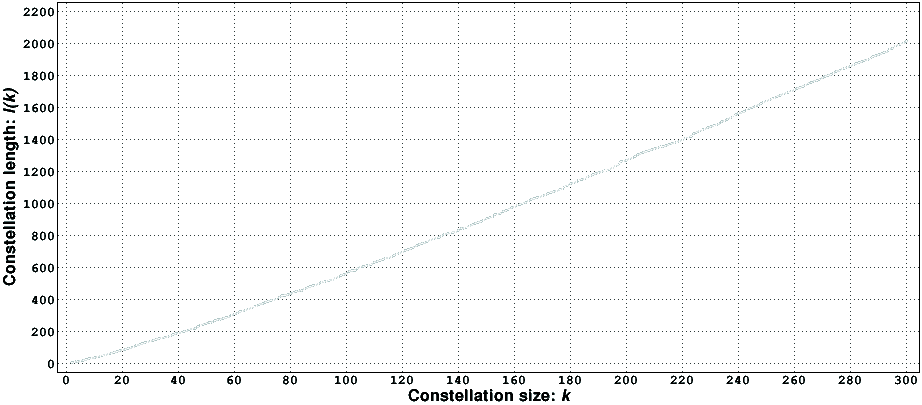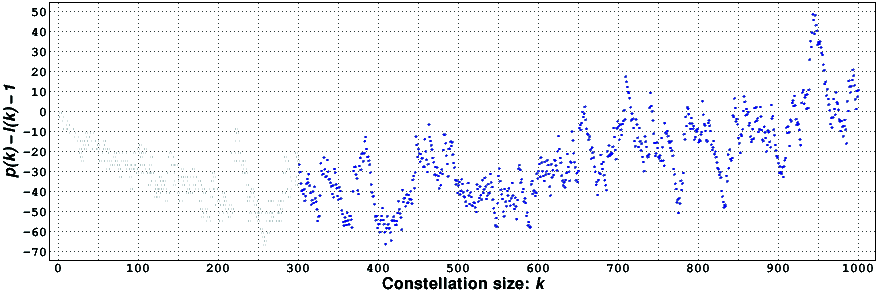## Introduction

Let a_1 < a_2 < ... < a_k be k distinct integers, sorted in increasing order. In a famous memoir , Hardy and Littlewood conjectured that there exists an infinite number of values of n for which the k integers n+a_1, n+a_2, ..., n+a_k are simultaneously prime, provided that there does not exist a prime number that divides, for all n, the integers (n+a_1)(n+a_2)...(n+a_k), or, what is the same, provided that for each prime number p there exists some congruence class (mod p) which contains none of the a_i. This conjecture is known as the prime k-tuple conjecture. Because n is arbitrary, we will assume, without loss of generality, that a_1=0, thus forcing all the a_i to be even numbers.

Let P(x;0,a_2,...,a_k) be the number of values of n not larger than x for which the integers n, n+a_2, ..., n+a_k are prime. Hardy and Littlewood also conjectured that when x tends to infinity P(x;0,a_2,...,a_k) tends asymptotically to

```                                              k-1
/  p  \    / p-v \
Q(x;0,a_2,...,a_k) = Li(x;k)  PRODUCT  | --- |    | --- | ,
p prime  \ p-1 /    \ p-1 /
```

where the product is over all the prime numbers p, where v is the number of distinct residues (mod p) of the numbers 0, a_2, ..., a_k, and where Li(x;k) is the generalized logarithmic integral, i.e., it is the integral of (log u)^(-k) with [2,x] as the interval of integration (sometimes zero is also used as the lower limit of integration, in which case one uses the principal value of the integral). When k=1 this formula becomes the well known asymptotic formula for the prime counting function pi(x). The numerical evidence supporting this conjecture is very strong (see chapter 3 of ).

The k-tuples (0,a_2,...,a_k) that satisfy the two equivalent conditions stated above are called admissible constellations; k is the size of the constellation and 1+a_k is its length. Thus, the length of the constellation is the number of consecutive integers in the interval [n,n+a_k], and the size of the constellation is the maximum number of primes it might have for a given value of n. Note that if v=p for some p in the asymptotic formula given above, then the constellation is not admissible.

Let s(x) be the largest possible size of an admissible constellation with length not larger than x, and let l(k) be the minimum length of an admissible constellation of size k. From these definitions it follows that s(x) is a piecewise-constant function (continuous on the right) that jumps from k-1 to k when x reaches l(k). We present below a graph of l(k). It is worthwhile to mention here that the first few values of l(k) can be found in the On-Line Encyclopedia of Integer Sequences as the sequence A020497.

In the same memoir , Hardy and Littlewood conjectured, based on empirical evidence, that rho(x) <= pi(x) for x >= 2, where rho(x) is the largest number of primes that occur indefinitely often in an interval of length x. Assuming the truth of the prime k-tuple conjecture, rho(x)=s(x). This second conjecture is connected with another conjecture of Hardy and Littlewood, which states that pi(x+y) - pi(x) <= pi(y) for x,y >= 2, i.e., it states that no interval with n>1 consecutive integers can contain more primes than the interval [1,n]. We will call this conjecture the pi(x) conjecture. In 1974, Hensley and Richards proved that the pi(x) conjecture is incompatible with the prime k-tuple conjecture (see  for a summary of their work, and [4, problem A9]). Since the prime k-tuple conjecture is believed to be true, the pi(x) conjecture is believed to be false. This is in accord with the numerical evidence gathered in the past, some of which we will present below. No actual counter-example of the pi(x) conjecture has so far been found, although some admissible constellations of length x with size larger than pi(x) -- which we call interesting constellations -- are known (see , where a constellation with size 1412 and length 11763 is mentioned, [5, problem 1.89], where an interesting constellation with length 4893 is mentioned, and , where an interesting constellation of size 458 and length 3243 is presented).

In order to actually find a counter-example of the pi(x) conjecture, the first step is to find an interesting constellation. Because Q(x;0,a_2,...,a_k) tends asymptotically to

```                    x
C(0,a_2,...,a_k) --------
log(x)^k
```

where C(0,a_2,...,a_k) is a non-zero constant for admissible constellations, to reduce the expected size of the first x that makes P(x;0,a_2,...,a_k) nonzero, it is crucial to either make C(0,a_2,...,a_k) as large as possible or to make k as small as possible. This expected size is also a good indication of the computational effort required to find a counter-example. Interesting constellations of small size are therefore needed to speedup the search for a counter-example of the pi(x) conjecture.

Admissible constellations also play an important role in recent important results about bounded gaps between primes , .

## Computational results (July 14, 2015)

In order to attempt to find an interesting admissible constellation of small size, we wrote a computer program that finds the minimum length, l(k), of an admissible constellation of size k. Unfortunately, the execution time of the program grows exponentially, and reaches times measured in days for constellations with sizes around 280. As a consequence, we were not able to find an interesting constellation of small size. Nonetheless, based on our results, we are able to present estimates of sizes of possibly interesting constellations; as detailed below, the sizes 384, 463, 658, and 709 appear to be promising. According to Thomas Engelsma , the size 458 has at least one interesting constellation.

The following figure presents a graph with the values of l(k) we were able to compute.Although the local rate of increase of l(k) exhibits some fluctuations, its global rate of increase appears to be very regular. In particular, it appears that k(1+log k) is a very good approximation to l(k), as shown in the following figure. It also appears that the absolute value of the error is bounded from above by the square root of k multiplied by a constant close to one (shaded area), at least for small values of k.This approximate formula for l(k) allows us to estimate the sizes of admissible constellations for which it is probable that s(x) > pi(x). Let p(k) be the k-th prime number. Among all the integer values of x for which pi(x) = k, i.e., those between p(k) and p(k+1)-1, the one that corresponds to the largest s(x) is x=p(k+1)-1. We are interested in finding a small k for which s(p(k+1)-1) > k. Since s(l(k)) = k, it follows that s(x) > k for x >= l(k+1). Combining the two inequalities yields p(k+1)-1 >= l(k+1). Thus, if p(k)-l(k)-1 >= 0 we have found an admissible constellation, of size k-1, which satisfies s(x) > pi(x) for x = l(k-1). In this table [12KiB, compressed with gzip] we present values of p(k) and of l(k), these last either computed or estimated, for k up to 1000. An analysis of this table shows that 384, 463, 658, and 709 are promising values for k. This can also be seen in the following figure (blue dots are estimates).For k up to around 200, p(k)-l(k)-1 has a tendency to decrease. From k around 200 up to k around 400, we estimate that the tendency of p(k)-l(k)-1 to decrease stops. Starting at k around 400, we estimate that p(k)-l(k)-1 has a tendency to increase, becoming always positive at k around 1300. If this prediction is correct, interesting constellations will be "abundant" for large k.

## References

  G. H. Hardy and J. E. Littlewood, Some problems of `partitio numerorum'; III: on the expression of a number as a sum of primes, Acta Mathematica, vol. 44, pp. 1-70, 1922.  Hans Riesel, Prime numbers and computer methods for factorization, second edition, Birkhäuser, 1994.  Ian Richards, On the incompatibility of two conjectures concerning primes; a discussion of the use of computers in attacking a theoretical problem, Bulletin of the American Mathematical Society, vol. 80, no. 3, pp. 419-438, May 1974.  Richard K. Guy, Unsolved problems in number theory, third edition, Springer-Verlag, 2004.  Richard Crandall and Carl Pomerance, Prime numbers: a computational perspective, second printing, Springer-Verlag, 2001.  Thomas J. Engelsma, k-tuple permissible patterns, web page, February 2005.  Yitang Zhang, Bounded gaps between primes, Annals of Mathematics, vol. 179, no. 3, pp. 1121-1174, 2014.  DHJ Polymath, Variants of the Selberg sieve, and bounded intervals containing many primes, Research in the Mathematical Sciences, vol. 1, no. 12, 83 pages, 2014. Erratum in vol. 2, no. 15, 2015.